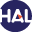# Volume 5 N°1 (2008)

Latest addition : 8 September 2010.

• ### Convergence analysis of an MPFA method

6 December 2009,

Full Title:

Convergence analysis of an MPFA method for flow problems in anisotropic heterogeneous porous media

Abstract:

Our purpose in this paper is to present the theoretical analysis of a Multi-Point Flux Approximation method (MPFA method).

We start with the derivation of the discrete problem, and then we give a result of existence and uniqueness of a solution for that problem. As in finite element theory, Lagrange interpolation is used to define three classes of continuous and locally polynomial approximate solutions. For analyzing the convergence of these different classes of solutions, the notions of weak and weak-star MPFA approximate solutions are introduced.

Their theoretical properties, namely stability and error estimates (in discrete energy norms, L2 norm and L(infinity) norm), are investigated. These properties play a key role in the analysis (in terms of error estimates for diverse norms) of different classes of continuous and locally polynomial approximate solutions mentioned above.Voir sur HAL : https://hal.archives-ouvertes.fr/hal-01120075

• ### Description of numerical shock profiles of non-linear Burgers’ equation by asymptotic solution of its differential approximations

8 February 2008,

Full Title:

Description of numerical shock profiles of non-linear Burgers’ equation by asymptotic solution of its differential approximations

Abstract:

An analysis of dispersive/dissipative features of the difference schemes used for simulations of the non-linear Burgers’ equation is developed based on the travelling wave asymptotic solutions of its differential approximation. It is shown that these particular solutions describe well deviations in the shock profile even outside the formal applicability of the asymptotic expansions, namely for shocks of moderate amplitudes. Analytical predictions may be used to improve calculations by suitable choice of the parameters of some familiar schemes, i.e., the Lax-Wendroff, Mac-Cormack etc. Moreover, an improvement of the scheme may be developed by adding artificial terms according to the asymptotic solution.Voir sur HAL : https://hal.archives-ouvertes.fr/hal-01127976